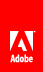# h

Determines the height of a given form design object.

#### Syntax

`Reference_Syntax.h( OBJECT param1 [, STRING param2 [, INTEGER param3 ] ] )`

#### Parameters

 param1 The fully qualified reference syntax expression of one of the following container XML Form Object Model objects: area , contentArea , draw , field , pageArea , subform . param2 (Optional) A string representing the unit type of the return value. If left blank, the default unit type is points. param3 (Optional) An integer representing a zero-based index value indicating the content area in which you want to obtain the object’s height. If left blank, the default value is 0 . This parameter allows you to calculate the height of an object that is distributed across multiple content areas, such as pages. For example, if you want to find the height of a subform object that spans multiple content areas, you would use this parameter to enumerate the height of the subform in each of the content areas and add the totals together.

#### Returns

The height of the form design object in the specified content area.

Model

Object

XFA 2.1

#### JavaScript

```// Returns the height of a single instance of TextField1
xfa.layout.h(TextField1,"in");

// Calculates the height of Subform1 across two content areas and displays
// the total in a message box.
var iHeight = xfa.layout.h(Subform1,"in",0) + xfa.layout.h(Subform1,"in",1);
xfa.host.messageBox(iHeight);```

#### FormCalc

```// Returns the height of a single instance of TextField1
xfa.layout.h(TextField1,"in")

// Calculates the height of Subform1 across two content areas and displays
// the total in a message box.
var iHeight = xfa.layout.h(Subform1,"in",0) + xfa.layout.h(Subform1,"in",1)
xfa.host.messageBox(iHeight)```// Ethnio survey code removed